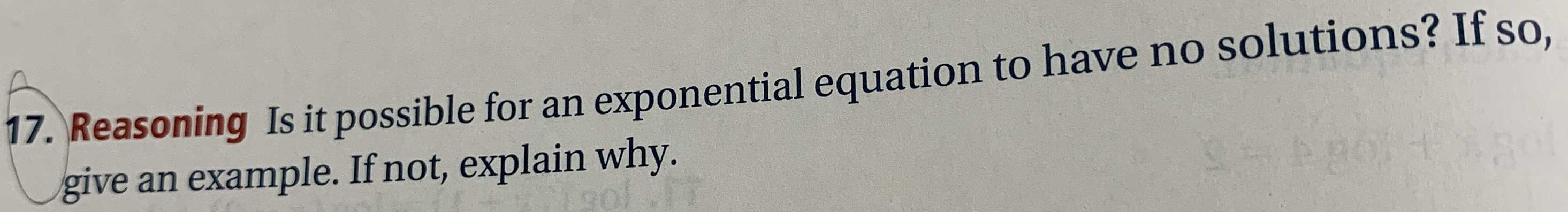### ¿Todavía tienes preguntas de matemáticas?

Pregunte a nuestros tutores expertos
Algebra
Pregunta17. Reasoning Is it possible for an exponential equation to have no solutions? If so, give an example. If not, explain why.

for example - 3$$^{x}$$=-1